﻿ Chemical Ordering in Na-Pb and Na-Hg Liquid AlloysAmerican Journal of Condensed Matter Physics

p-ISSN: 2163-1115    e-ISSN: 2163-1123

2017;  7(3): 67-72

doi:10.5923/j.ajcmp.20170703.02### Chemical Ordering in Na-Pb and Na-Hg Liquid Alloys

Ajayi Ayodeji Akintunde, Ogunmola Enoch Debayo, Adeoye Abiodun Eyitayo

Department of Mathematical and Physical Sciences, Afe Babalola University, Ado-Ekiti, Nigeria

Correspondence to: Ogunmola Enoch Debayo, Department of Mathematical and Physical Sciences, Afe Babalola University, Ado-Ekiti, Nigeria.
 Email: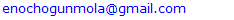Abstract

A simple model has been used to investigate the nature of chemical order in Na-Pb and Na-Hg liquid binary alloy at 700K and 673K respectively. The energy parameter obtained from the model was used to calculate the concentration dependent mixing properties such as Gibb’s free energy of mixing, Concentration fluctuations in the long wavelength limit and the Warren-Cowley chemical short range order parameter. Results obtained showed that both alloys are hetero-coordinated throughout the entire concentration and there is tendency for segregation and demixing to take place in the liquid alloys. We observed that Na-Hg liquid alloy is more strongly interacting binary alloy and chemically ordered than Na-Pb liquid alloy.

Keywords: Binary liquid alloy, Chemical ordering, Sodium, Theoretical study, Warren-Cowley parameter

Cite this paper: Ajayi Ayodeji Akintunde, Ogunmola Enoch Debayo, Adeoye Abiodun Eyitayo, Chemical Ordering in Na-Pb and Na-Hg Liquid Alloys, American Journal of Condensed Matter Physics, Vol. 7 No. 3, 2017, pp. 67-72. doi: 10.5923/j.ajcmp.20170703.02.

### 1. Introduction

A number of theories have been developed by theoreticians to explain the temperature dependence of the thermodynamic properties of binary liquid alloys with a purpose of obtaining valuable microscopic information on them [1-3]. Accurate thermodynamic knowledge of the alloy mixing properties and phase diagrams of alloy systems is crucial to having a reliable theoretical result. The mixing behavior of two metals forming binary alloys is as a result of the interplay between energetic and structural adjustments of constituent elemental atoms . Hetero-coordination known as the preference of unlike atoms to pair as nearest neighbors in the alloy system, form A-B pair while segregation is known as the situation where the constituent atoms in the alloy becomes self-coordinated forming A-A and B-B pairs where A and B are the constituent atoms within the binary alloy . Alloys are mixture of metals and a binary alloy can be represented as AxB1-x where A and B are metals and subscripts x and 1-x are concentration of the respective metals. Na-Pb, Na-Hg, K-Pb and K-Hg are examples of binary alloys. Classification of binary alloys can be done according to the deviation of their thermodynamic functions such as chemical activity from raoulatian ideality, therefore binary alloys can be classified into two main groups; segregating (positive deviating) and heteoro-coordinating (negative deviating) alloys [6, 7]. The interest in the energetic and its effects on the alloying behavior of Na-Pb and Na-Hg stemmed from the understanding that, despite the hazards involved in the unsafe handling of Na-Pb alloy, it may still be relevant in qualitative inorganic analysis, both as reductant in acidic and alkaline media, and also as a source of sodium hydroxide for hydroxide precipitations . Na-Hg has been used in organic chemistry as a powerful reducing agent, which is safer to handle than sodium itself. Na-Hg has been found to be useful in fabricating pressure-based sodium lamp . It is necessary to mention that there has been a previous attempt to understand the alloying behavior of these alloys . Consequently, this paper also has the purpose of complimenting earlier studies on each of Na-Pb and Na-Hg liquid alloy by studying the structural behaviour of these two Sodium-based alloy systems, mostly with, studies of concentration fluctuations and the Warren-Cowley chemical short-range order parameter (CSRO). The calculation of the structural properties using Flory’s model  is presented.

### 2. Theoretical Concepts

In the framework of the Flory’s model, the expression for the Gibb’s free energy of mixing,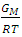, of a binary liquid alloy is given by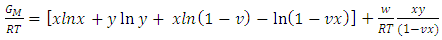(1)
Where in Eqn.1, x and y are the bulk concentrations of the constituent A and B atoms in the binary alloy respectively such that, y=1-x, and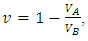VA and VB being atomic volumes of constituents’ A and B respectively. The parameter w is the ordering energy whose value gives information on the alloying behaviour of the alloy. R is a universal gas constant.
The concentration fluctuations in the long-wavelength limit, Scc(0) can be calculated from the standard relationship in terms of the Gibbs free energy of mixing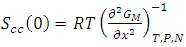(2)
Using equations (1), (2), Scc(0) becomes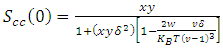(3)
Where;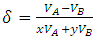(4)
For ideal mixing, the energy parameters w and δ are zero and eqn. (3) reduces to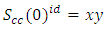(5)

### 3. Results and Discussion

#### 3.1. Free Energy of Mixing

Table 1 shows the values of the fitted interaction parameter for liquid Na-Pb and Na-Hg. Eqn. (1) has been used in obtaining, the optimal value for the interaction parameter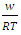that gives agreement between experimental and theoretical Gibbs free energy. Eqn. (1) was also used to calculate the GM/RT for both systems while the experimental data were taken from the work reported by Hultgren et. al., . Figure 1 and 2 shows the computed and experimental values as a function of concentration. The negative values of w in Table 1 show both alloy systems are chemically ordered, which means pairing of unlike atoms. From the results in Figure 1 and 2, it could be seen that both systems Na-Pb and Na-Hg have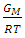minimum values of -2.797 and -3.125 respectively. This is an indication that Na-Hg is more heterocoordinated than Na-Pb or strongly interacting binary alloy,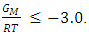Table 1. Fitted parameters for Na-Pb and Na-Hg liquid alloys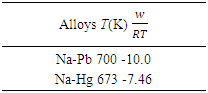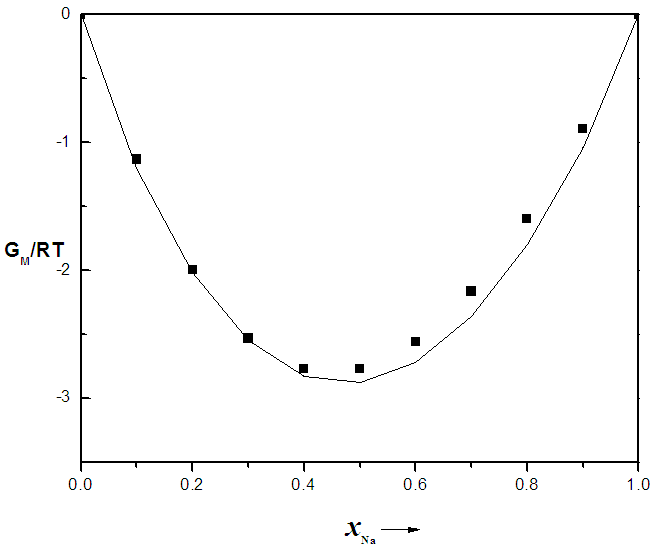Figure 1. Concentration dependence of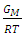for Na-Pb liquid alloys at 700K, computed from Eqn.1. The solid line represents theoretical values and the squares represent experimental values , xNa is the concentration of Na in the alloy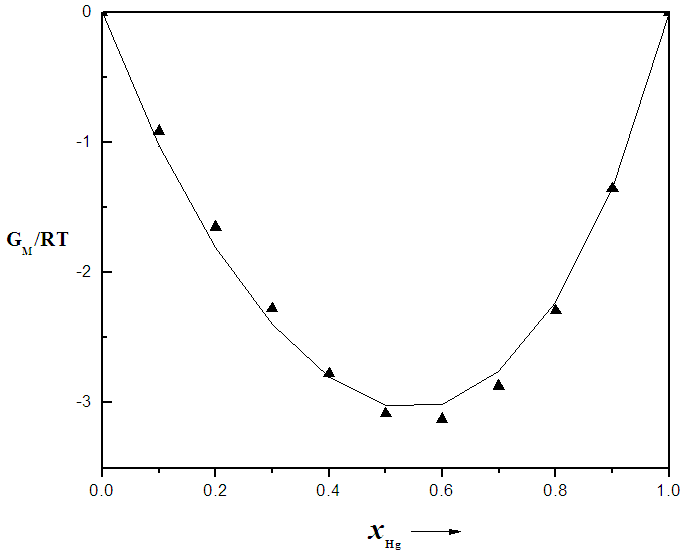Figure 2. Concentration dependence of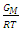for Na-Hg liquid alloys at 673K, computed from Eqn.1. The solid line represents theoretical values and the squares represent experimental values . xHg is the concentration of Hg in the alloy

#### 3.2. Concentration Fluctuations and the Warren-Cowley CSRO Parameter

The fitted parameters were kept invariant when computing Scc(0) and the short-range order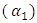. In principle, Scc(0) can be obtained directly from small-angle diffraction experiments but the experimental procedure involved is very tedious and has not been accomplished successfully. The Scc(0) is an essential microscopic parameter which has been widely used in the study of nature of atomic order in binary liquid alloys [13, 14] and the Scc(0) with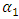and it also formed a basis for explaining energetics in liquid alloys. Figure 3 and 4 shows the computed values of the concentration fluctuations in the long wavelength limit. Eqs. (3) and (5) has been used to compute Scc(0) and Scc(0)id respectively. The mixing behavior of liquid binary alloys can be deduced from the deviation of Scc(0) from the ideal value. Scc(0) < Scc(0)id is an evidence of chemical ordering and heterocoordination otherwise, there is tendency for segregation and demixing to take place in the liquid alloys. Fig. 3 and 4 shows heterocoordination in both alloys.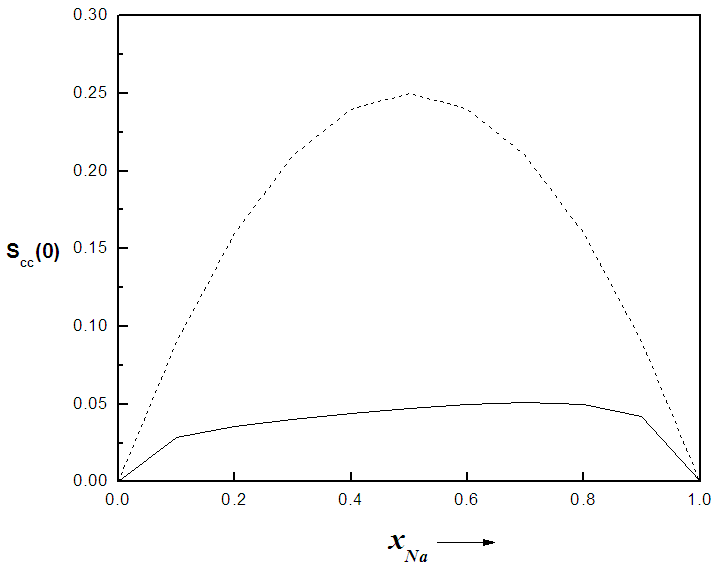Figure 3. Concentration fluctuations in the long wavelength limit, real (Scc(0)) and ideal (Scc(0)id) versus concentration for Na-Pb system at 700K computed from Eqn. 3 and 5. The solid line represent Scc(0) and the dashes represent the values of Scc(0)id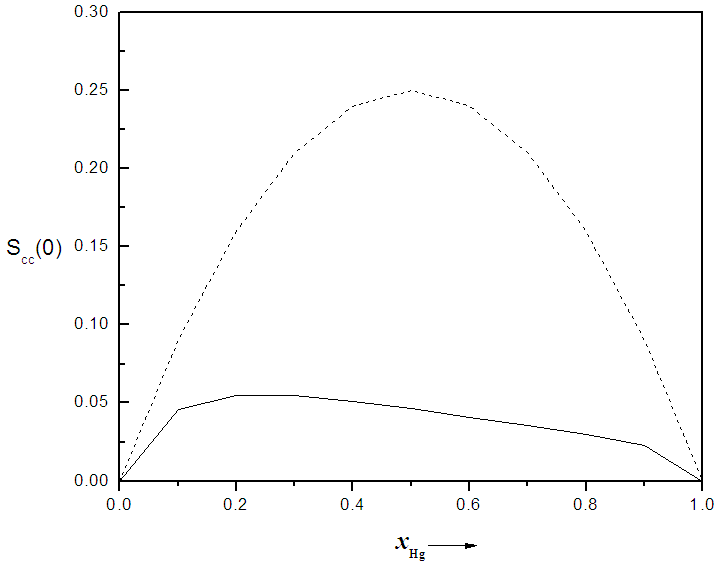Figure 4. Concentration fluctuations in the long wavelength limit real (Scc(0)) and ideal (Scc(0)id) versus concentration for Na-Hg system at 673K computed from Eqn. 3 and 5. The solid line represent theoretical values and the dashes represent the values of Scc(0)id
The nature of ordering in binary liquid alloys can also be investigated by calculating the Warren Cowley Short Range Order (CSRO) parameter, α1 [4, 5]. Experimental values are not available to compare our theoretical results just as we have done in the case of the Gibb’s free energy of mixing. Scc(0) and α1 are related by the expression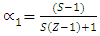(6)
Where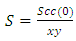(7)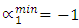indicates complete ordering (unlike atoms pairing) while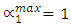represent segregation. The degree of chemical order may be deduced from the negative values of α1. Maximum heterocoordination can be observed at xNa = 0.41 in Na-Pb and at xHg = 0.72 in Na-Hg as depicted by figures 5 and 6. α1 for Na-Pb at xNa = 0.41 is -0.30 and in the case of NaHg at xHg = 0.72 is -0.34. This reveals Na-Hg has more heterocoordination and chemical order than Na-Pb.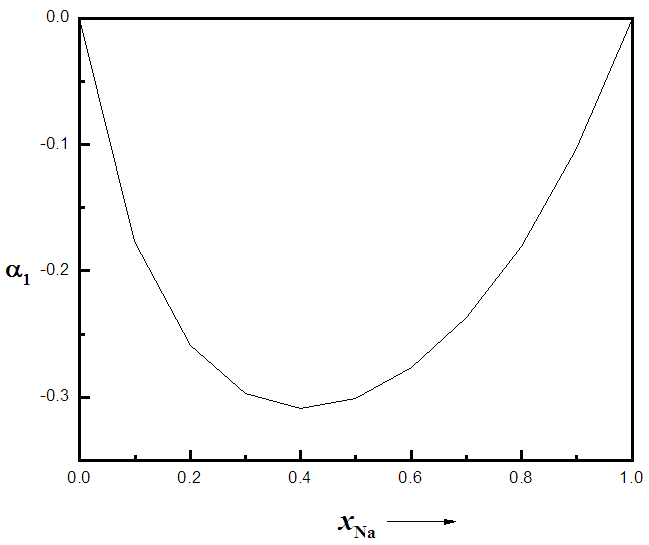Figure 5. Calculated Warren-Cowley CSRO parameter, α1, using eqn. (6) for Na-Pb at 700K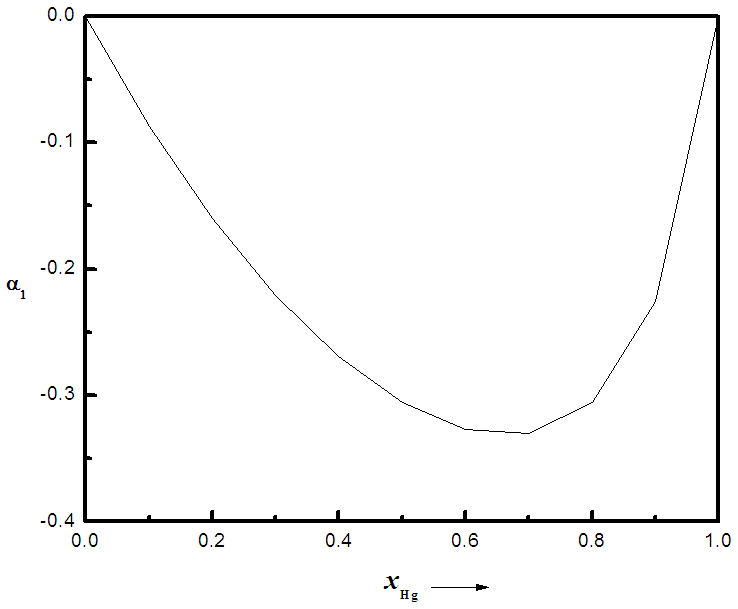Figure 6. Calculated Warren-Cowley CSRO parameter, α1, using eqn. (6) for Na-Hg at 673K

### 4. Conclusions

The energetics of Na-Pb and Na-Hg liquid binary alloys have been analyzed in this present study at 700K and 673K respectively. Attention has been given to their thermodynamic functions such as Gibb’s free energy of mixing, concentration fluctuations and Warren-Cowley CSRO parameter. Theoretical study of the alloying behavior of the two liquid alloys reveals hetero-coordination in both alloys throughout the entire concentration of Na in Na-Pb and Hg in Na-Hg. It has also been shown that Na-Hg alloy is a more strongly interacting binary alloy than Na-Pb.

### References

  Bhatia, A.B. and Hargrove, W.H. (1974). Concentration fluctuations and thermodynamic properties of some compound forming binary molten systems, Physical Review B, 10, 3186.  Singh, N.K.P., Singh, R.N. and Choudary, R.B. (1987). Thermodynamic investigation of atomic order in Al-Mg liquid alloys, Journal of Physics: Condensed Matter, 3, 20.  Akinlade, O. (1995). Ordering phenomenon in Na-Ga and Na-Sn molten alloys, Physics and Chemistry of Liquids, 29, 9-21.  Akinlade, O., Singh, R.N. (2001). Correlation between bulk and surface phenomenon in Ga-(Bi,In) and In-Bi liquid alloy, International Journal of Physics B, 15, 22.  Odusote, Y.A. (2008). Investigation of ordering phenomenon in Me-Pt (Me=Fe,Ni) liquid alloys, Science and Technology of Advanced Materials, 9,1-7.  Anusionwu, B.C. and Adebayo, G.A. (2001). Quasi-chemical studies of ordering in the Cu-Zr and Cu-Si melts, Journals of Alloys and Compounds, 329, 162-167.  Odusote, Y.A., Hussain, L., and Awe, O.E. (2007). Bulk and dynamic properties of Al-Zn and Bi-In liquid alloys using a theoretical model, Journals of Non-Crystalline Solids, 353, 1167-71.  Robert, A.E. and Gerald, W.A. (1965). Applications of sodium-lead alloy in qualitative inorganic analysis, Journal of Chemical Education, 42, 436.  Greenwood, N.N. and Earnshaw, A. (2007). Chemistry of elements (Second edition), Butterworth – Heinemann.  Singh, B.P., Kumar, J., Jha, I.S. and Adhikari, D. (2011). Concentration dependence of thermodynamic properties of NaPb liquid alloy, World Journal of Condensed Matter Physics, 1, 97-100.  Flory, P.J. (1942). Thermodynamics of high-polymer solutions, Journal of Chemical Physics, 10, 51-61.  Alonso, J.A., Lopez, J.M., and March, N.H. (1982). Concentration fluctuations in simple liquid alloys with atomic size mismatch. Journal de Physique Lettres, 43 (12), pp.441-445.  Hultgren, R., Desai, P.D., Hawking, D.T., Gleiser, M. and Kelley, K. (1973). Selected values of the thermodynamic properties of binary liquid alloys, Metal Park: ASM, OH.  Alonzo, J.A., Lopez, J.M., and March, N.H. (1982). Concentration fluctuations in simple liquid alloys with atomic size mismatch, Journal of Physique Lettres, 43, 441-445.